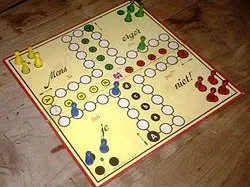Get the Arduino Dice © GPL3+

• 2,484 views
• 6 respects

Apps and online services

Don't trust your cubed dice? You believe your teammates in "Mensch ärgere Dich nicht" (roughly translated: Man does not annoy you) always have a six-sided dice at hand? Just let the random number generation work on your Arduino and display the number on a 1-digit, 7-segment display. The circuit is quickly assembled and should lead to a fair outcome of the board game.

Here is the mapping:

You can of course replace the delay of 1 second with a button, so that a new edition actually appears at the touch of a button.

If you do not know the board game yet, I have a link for you here.

Code

Random CubeC/C++
v1.0
// Arduino 7 segment display example software
// http://www.hacktronics.com/Tutorials/arduino-and-7-segment-led.html

int randNumber;

// Define the LED digit patters, from 0 - 9
// Note that these patterns are for common cathode displays
// For common anode displays, change the 1's to 0's and 0's to 1's
// 1 = LED on, 0 = LED off, in this order:

// Arduino pin: 2,3,4,5,6,7,8
byte seven_seg_digits =
{
{ 1,1,1,1,1,1,0 },  // = 0
{ 0,1,1,0,0,0,0 },  // = 1
{ 1,1,0,1,1,0,1 },  // = 2
{ 1,1,1,1,0,0,1 },  // = 3
{ 0,1,1,0,0,1,1 },  // = 4
{ 1,0,1,1,0,1,1 },  // = 5
{ 1,0,1,1,1,1,1 },  // = 6
{ 1,1,1,0,0,0,0 },  // = 7
{ 1,1,1,1,1,1,1 },  // = 8
{ 1,1,1,0,0,1,1 }   // = 9
};

void setup() {
pinMode(2, OUTPUT);
pinMode(3, OUTPUT);
pinMode(4, OUTPUT);
pinMode(5, OUTPUT);
pinMode(6, OUTPUT);
pinMode(7, OUTPUT);
pinMode(8, OUTPUT);
pinMode(9, OUTPUT);
}

void writeDot(byte dot) {
digitalWrite(9, dot);
}

void sevenSegWrite(byte digit) {
byte pin = 2;
for (byte segCount = 0; segCount < 7; ++segCount) {
digitalWrite(pin, seven_seg_digits[digit][segCount]);
++pin;
}
}

void loop() {
// print number from 9 to 1
//  for (byte count = 10; count > 0; --count) {
//   delay(1000);
//   sevenSegWrite(count - 1);

// print a random number from 1 to 6
randNumber = random(1, 7);
Serial.println(randNumber);
sevenSegWrite(randNumber);

delay(1000);
}

Arduino Dice

Project tutorial by RoboCircuits

• 6,773 views
• 24 respects

LED Dice

Project showcase by EvdS

• 80,855 views
• 187 respects

Settlers Of Catan Dice

Project tutorial by joshi

• 9,148 views
• 32 respects

Digital Dice

Project showcase by SKYMDev

• 5,310 views
• 7 respects